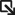• Solutions Solutions
• Products Products
• Services Services
• Support SupportSupport Portal ›
• Resources Resources
##### Networking Made Simple, Easy, and Affordable - Q&A with the Senior Director of Extreme Capital Solutions
All stories ›
• Partners Partners

# Delta-Ratio Expression

A CLEAR-Flow delta-ratio expression is a combination of the delta and ratio expressions.

The CLEAR-Flow agent computes the difference from one sample to the next for each of the two counters. The ratio of the differences is then compared to the threshold value. The following is the syntax for a CLEAR-Flow delta-ratio expression (note the similarity to the delta expression):

```delta-ratio <counterNameA> <counterNameB> REL_OPER <countThreshold> ;
min-value <min-value> ;
hysteresis <hysteresis> ;
```

The values of countThreshold and hysteresis can be specified as floating point numbers, and the delta-ratio is computed as a floating point number. The delta-ratio statement specifies how to compare the ratio of the counter differences with its threshold. The difference of the sample values of counterNameA is divided by the difference of the sample values of counterNameB, to compute the ratio that is compared with the countThreshold. The REL_OPER is selected from the relational operators for greater than, greater than or equal to, less than, or less than or equal to (>, >=, <, <=).

The min-value statement is optional and sets a minimum value for the counters. If either counter is less than the minimum value, the expression evaluates to false. If not specified, the minimum value is 1.

The hysteresis hysteresis statement is optional, and sets a hysteresis value for the threshold. After the ratio statement is true, the value of the threshold is adjusted so that a change smaller than the hysteresis value will not cause the statement to become false. For statements using the REL_OPER > or >=, the hysteresis value is subtracted from the threshold; for < or <=, the hysteresis value is added to the threshold.

For example, the following delta-ratio expression:

```delta-ratio counter1 counter2 >= 5 ;
min-value 100 ;
hysteresis 1 ;
```

will only be true after the ratio of the deltas of the counters reached at least 5. At the time it became true, the hysteresis value would be subtracted from the threshold (setting the threshold to 4). With the threshold now at 4, the condition would stay true until the ratio of the deltas of the counters became less than 4.

If the statement becomes false, the threshold is reset to its original value. You can use the hysteresis value to prevent the rule from vacillating between the true and false states if the ratio of the deltas of the counters is near the threshold. If the hysteresis value is greater than the threshold value, the hysteresis value will be set to 0.

The following table is an example of evaluating the CLEAR-Flow delta-ratio expression above multiple times. Notice that the rule is not triggered at the second evaluation because both counters have not yet reached the min-value of 100. The rule first triggers at evaluation 4, when ratio of the two counters exceeds 5. After the rule is triggered, it remains triggered until the ratio value is less than 4 (the original threshold minus the hysteresis), at evaluation 6. At evaluation 8, the rule is again triggered when the ratio reaches 5. The rule will remain triggered until the ratio drops below 4.Delta-Ratio Expression Evaluation Example

Evaluation counter1 value counter1 delta counter2 value counter2 delta Ratio Rule Triggered?
1 110 N/A 20 N/A N/A No
2 537 427 90 70 6 No
3 1478 941 325 235 4 No
4 3953 2475 737 412 6 Yes
5 6261 2308 1307 570 4 Yes
6 8574 2313 2078 771 3 No
7 12171 3597 2977 899 4 No
8 17511 5340 4042 1065 5 Yes

See Delta-Ratio Expression Example for a full example of an ACL (Access Control List) and a CLEAR-Flow rule using a delta-ratio expression.